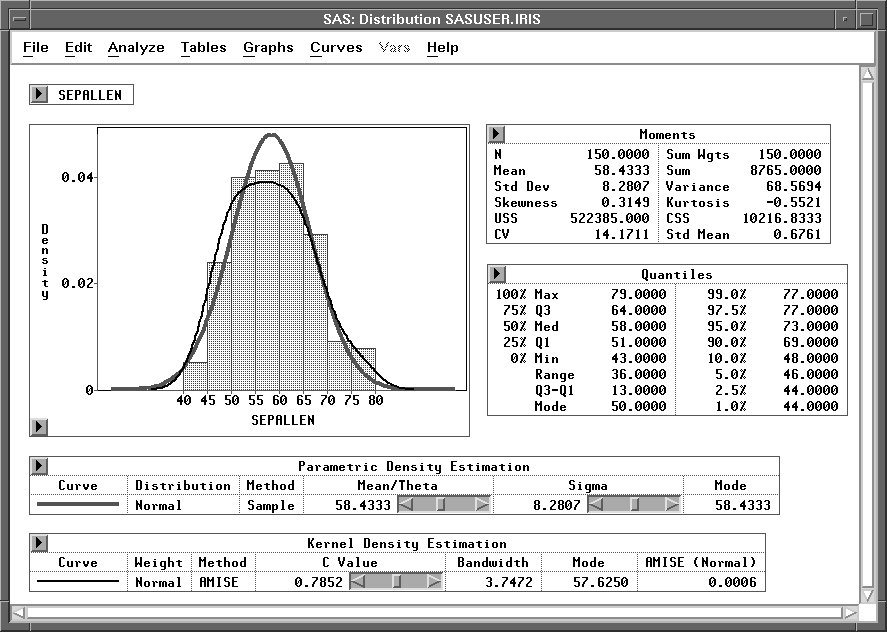Previous Page Next Page
 Distribution Analyses

# Distribution Analyses

Choosing Analyze:Distribution ( Y ) gives you access to a variety of distribution analyses. For nominal Y variables, you can generate bar charts, mosaic plots, and frequency counts tables.

For interval variables, you can generate univariate statistics, such as moments, quantiles, confidence intervals for the mean, standard deviation, and variance, tests for location, frequency counts, robust measures of the scale, tests for normality, and trimmed and Winsorized means.

You can use parametric estimation based on normal, lognormal, exponential, or Weibull distributions to estimate density and cumulative distribution functions and to generate quantile-quantile plots. You can also generate nonparametric density estimates based on normal, triangular, or quadratic kernels.

You can use Kolmogorov statistics to generate confidence bands for the cumulative distribution and to test the hypothesis that the data are from a completely specified distribution with known parameters. You can also test the hypothesis that the data are from a specific family of distributions but with unknown parameters.Figure 38.1: Distribution Analysis

#### References

 Previous Page Next Page Top of Page